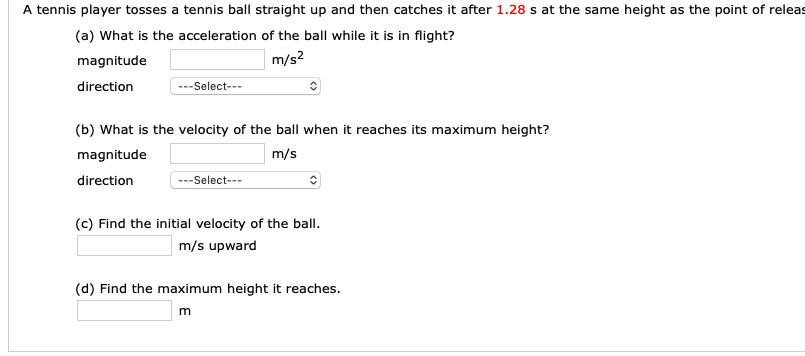# A tennis player tosses a tennis ball straight up and then catches it after 1.28 s at the same height as the point of releas(a) What is the acceleration of the ball while it is in flight?magnitudedirectionm/s2--Select--(b) What is the velocity of the ball when it reaches its maximum height?magnitudedirectionm/s-Select---(c) Find the initial velocity of the ballm/s upward(d) Find the maximum height it reaches.

Question
2862 viewshelp_outlineImage TranscriptioncloseA tennis player tosses a tennis ball straight up and then catches it after 1.28 s at the same height as the point of releas (a) What is the acceleration of the ball while it is in flight? magnitude direction m/s2 --Select-- (b) What is the velocity of the ball when it reaches its maximum height? magnitude direction m/s -Select--- (c) Find the initial velocity of the ball m/s upward (d) Find the maximum height it reaches. fullscreen
check_circle

Step 1

Part (a):

When the ball is thrown upwards the only force acting on it is force of gravitation. Thus, the acceleration of the ball is acceleration due to gravity. The direction of acceleration is downwards due to effect of gravitational force and its magnitude is same as acceleration due to gravity.

Step 2

Part (b):

When the ball is moving upward the acceleration is decreasing with increase in height. At maximum height the ball stops and starts moving downwards. Thus, the velocity of the tennis ball at maximum height is zero. The direction of the velocity will be downward at maximum height because after this point the ball will start moving downwards.

v = 0 m/s in downward direction

Step 3

Part (c):

When the tennis ball is moving in upward direction to maximum height. Initial velocity of the ...

### Want to see the full answer?

See Solution

#### Want to see this answer and more?

Solutions are written by subject experts who are available 24/7. Questions are typically answered within 1 hour.*

See Solution
*Response times may vary by subject and question.
Tagged in

### Physics